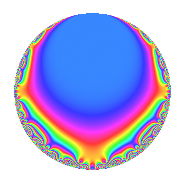# Properties

 Label 41.2.bLevel 41 Weight 2 Character orbit b Rep. character $$\chi_{41}(40,\cdot)$$ Character field $$\Q$$ Dimension 2 Newforms 1 Sturm bound 7 Trace bound 0

# Related objects

## Defining parameters

 Level: $$N$$ = $$41$$ Weight: $$k$$ = $$2$$ Character orbit: $$[\chi]$$ = 41.b (of order $$2$$ and degree $$1$$) Character conductor: $$\operatorname{cond}(\chi)$$ = $$41$$ Character field: $$\Q$$ Newforms: $$1$$ Sturm bound: $$7$$ Trace bound: $$0$$

## Dimensions

The following table gives the dimensions of various subspaces of $$M_{2}(41, [\chi])$$.

Total New Old
Modular forms 4 4 0
Cusp forms 2 2 0
Eisenstein series 2 2 0

## Trace form

 $$2q - 2q^{2} - 2q^{4} + 4q^{5} + 6q^{8} - 10q^{9} + O(q^{10})$$ $$2q - 2q^{2} - 2q^{4} + 4q^{5} + 6q^{8} - 10q^{9} - 4q^{10} - 2q^{16} + 10q^{18} - 4q^{20} + 16q^{21} - 2q^{25} - 16q^{31} - 10q^{32} - 16q^{33} + 10q^{36} + 4q^{37} + 32q^{39} + 12q^{40} - 6q^{41} - 16q^{42} + 8q^{43} - 20q^{45} - 2q^{49} + 2q^{50} - 16q^{57} + 8q^{59} - 4q^{61} + 16q^{62} + 14q^{64} + 16q^{66} - 30q^{72} + 28q^{73} - 4q^{74} + 16q^{77} - 32q^{78} - 4q^{80} + 2q^{81} + 6q^{82} - 24q^{83} - 16q^{84} - 8q^{86} + 32q^{87} + 20q^{90} - 32q^{91} + 2q^{98} + O(q^{100})$$

## Decomposition of $$S_{2}^{\mathrm{new}}(41, [\chi])$$ into irreducible Hecke orbits

Label Dim. $$A$$ Field CM Traces $q$-expansion
$$a_2$$ $$a_3$$ $$a_5$$ $$a_7$$
41.2.b.a $$2$$ $$0.327$$ $$\Q(\sqrt{-2})$$ None $$-2$$ $$0$$ $$4$$ $$0$$ $$q-q^{2}+\beta q^{3}-q^{4}+2q^{5}-\beta q^{6}-\beta q^{7}+\cdots$$